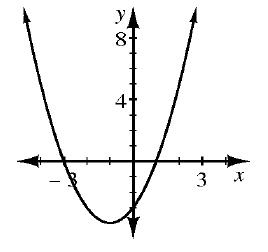Home > CCAA8 > Chapter cca9 > Lesson cca9.1.3 > Problem9-29

9-29.

Find the equation that represents the information given below. Homework Help ✎

1.If $y=(x−5)(x+7)$, then the $x$-intercepts of the graph are $(5,0)$ and $(−7,0)$.
Use the $x$-intercepts of each representation to write your equations.

$y=(x+3)(x−1)$

1.  $x$ $−4$ $−3$ $−2$ $−1$ $0$ $1$ $2$ $3$ $4$ $y$ $12$ $5$ $0$ $−3$ $−4$ $−3$ $0$ $5$ $12$